Contemporary Mathematics

# Chapter Review

### Chapter Review

##### Graph Basics
For the following exercises, use the given figure.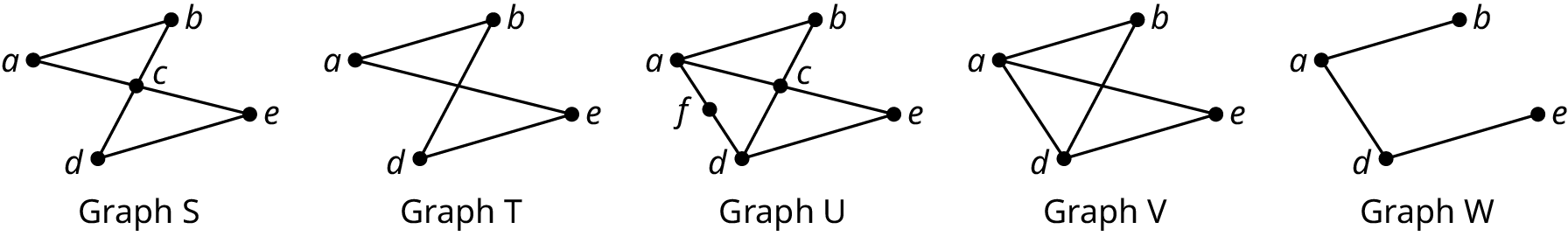1 .
Determine the number of vertices in Graph S.
2 .
Identify the graph with the fewest edges.
3 .
Name the vertices in Graph U.
4 .
Identify any pairs of vertices in Graph S that are not adjacent.
5 .
Which graphs only has vertices of degree 2?
6 .
Identify the graph in which the sum of the degrees of the vertices is 16.
7 .
Amazon.com has a network of warehouses that are used to move packages around the United States. Delivery trucks from warehouse deliver packages to other locations. These mail trucks also pick up packages to bring back to their home warehouse. Explain how a graph or multigraph might be drawn to model this scenario by identifying the objects that could be represented by vertices and the connections that could be represented by edges. Indicate whether a graph or a multigraph would be a better model.
8 .
Eduardo has two groups of four friends, group A and group B. Within each group, each of the members of the group are friends with each other but not with those in the other group. The individuals in group A have no other friends, but the individuals in group B each has their own group of four friends, and the individuals within those groups are all friends with each other, but with no one outside their own group. Draw a graph to represent the given scenario.
##### Graph Structures
For the following exercises, use the given figure.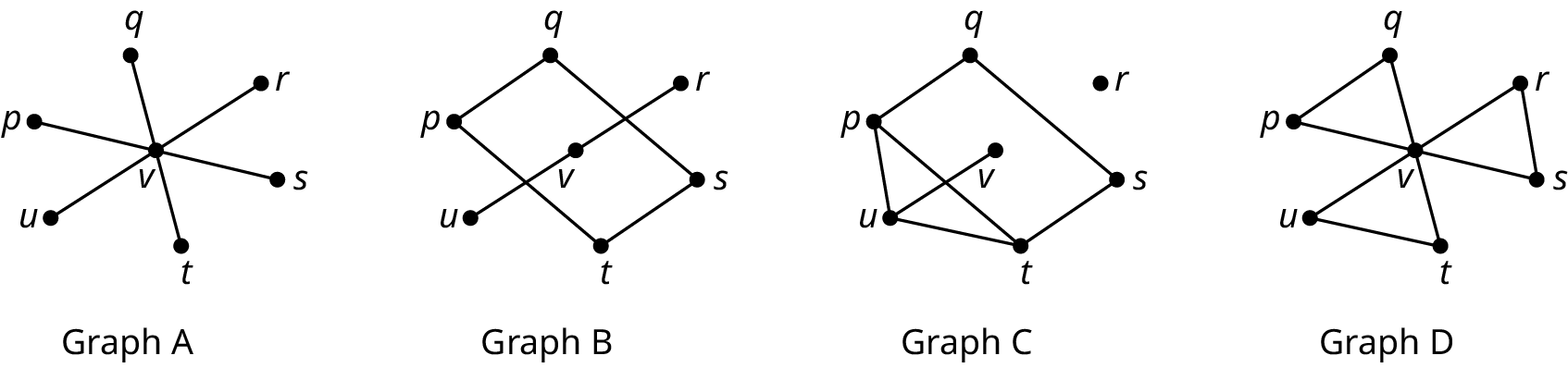9 .
Explain why Graph B is not a subgraph of Graph C.
10 .
Identify any graphs that have a quadrilateral cyclic subgraph and name the vertices.
11 .
Identify a clique in Graph C by listing its vertices.
12 .
Draw a graph with the following characteristics: largest clique has 4 vertices, a pentagonal cyclic subgraph, exactly two vertices of degree 4.
13 .
How many edges are in a complete graph with 12 vertices?
14 .
How many triangles are in a complete graph with 11 vertices?
##### Comparing Graphs
15 .
Identify three differences between Graph 1 and Graph 2 that demonstrate the graphs are not isomorphic.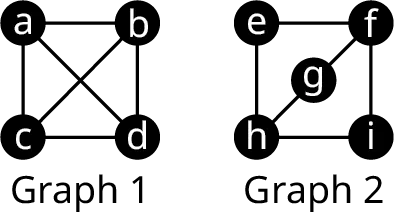For the following exercises, use the given figure.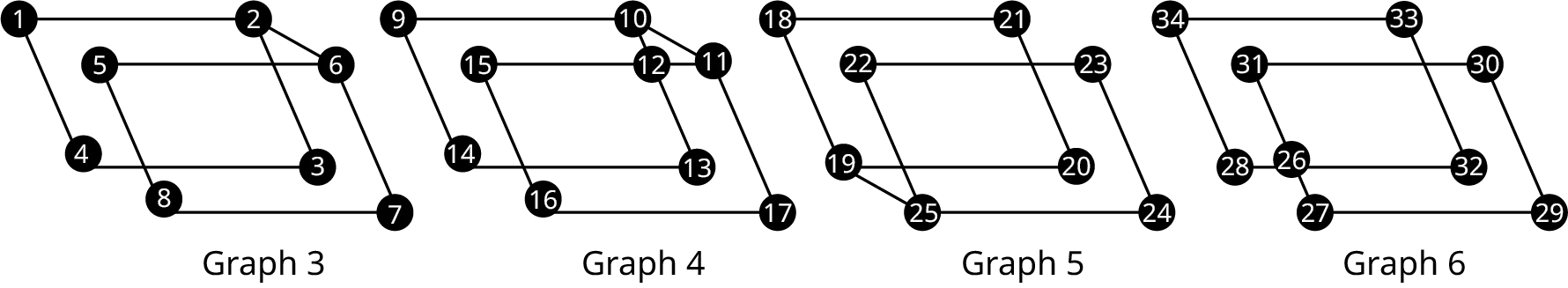16 .
Determine if Graph 3 is isomorphic to Graph 4. If so, identify a correspondence between the vertices which demonstrates the isomorphism. If not, identify at least two characteristics that verifies this.
17 .
Determine if Graph 3 is isomorphic to Graph 5. If so, identify a correspondence between the vertices that demonstrates the isomorphism. If not, identify a characteristic that verifies this.
18 .
Consider Graph 4 and Graph 5. Determine if one graph is a subgraph of the other. If so, give a correspondence between vertices that demonstrates this relationship. If not, identify conflicting characteristics.
For the following exercises, use the given figure.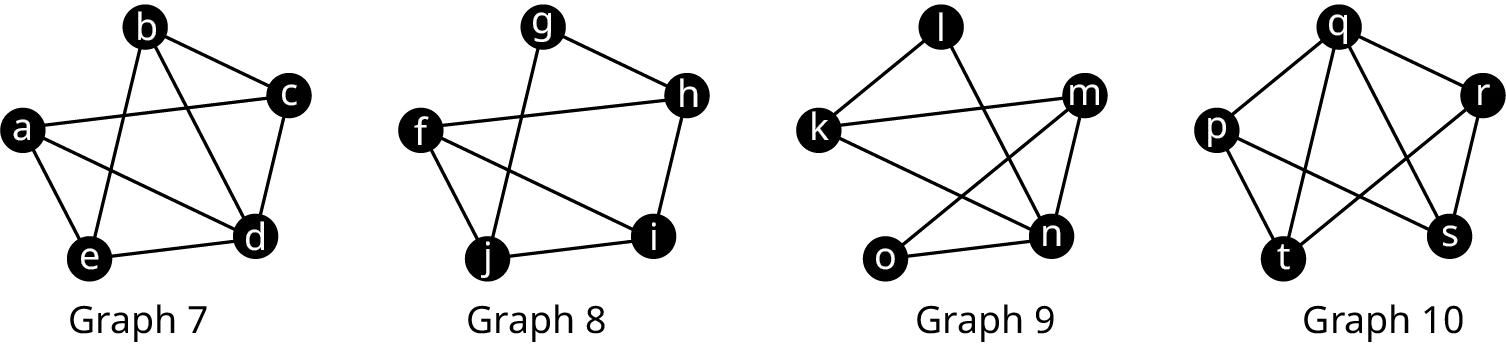19 .
Draw the complements of Graphs 7 and 9. Determine whether the graphs you drew are isomorphic to each other and explain how you know. Use this information to determine whether Graphs 7 and 8 are isomorphic.
20 .
Draw the complements of Graphs 7 and 10. Determine whether the graphs you drew are isomorphic to each other and explain how you know. Use this information to determine whether Graphs 7 and 10 are isomorphic.
21 .
Draw the complements of Graphs 8 and 9. Determine whether the graphs you drew are isomorphic to each other and explain how you know. Use this information to determine whether Graphs 8 and 9 are isomorphic.
##### Navigating Graphs
For the following exercises, use Graph A in the given figure. Consider each sequence of vertices. Determine if it is only a walk, both a walk and a path, both a walk and a trail, all three, or none of these.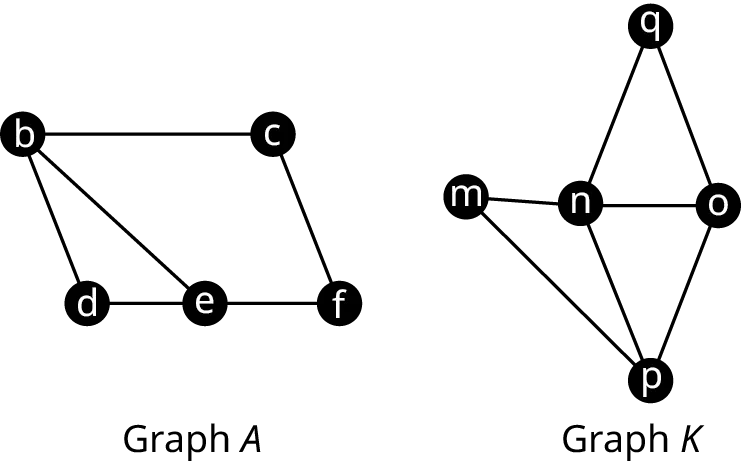22 .
edbef
23 .
ebdefcbd
24 .
efbd
For the following exercises, use Graph K in Figure 12.352. Identify each sequence of vertices as a closed walk, circuit (closed trail), and/or directed cycle (closed path). Indicate all that apply.
25 .
noqnpmn
26 .
mnoqnponm
27 .
poqnmp
For the following exercises, use Graphs A and K in Figure 12.352.
28 .
Are Graphs A and K planar graphs? What does your answer tell you about the chromatic number of each graph?
29 .
How many vertices are in the largest complete subgraph of Graph A? What does this tell you about the chromatic number of Graph A?
30 .
Create a coloring of Graph A, which uses exactly four colors, or explain why it is not possible.
31 .
Create a coloring of Graph K, which uses exactly two colors, or explain why it is not possible.
32 .
Determine the chromatic number of Graph A. Give a coloring that supports your conclusion.
33 .
Determine the chromatic number of Graph K. Give a coloring that supports your conclusion.
##### Euler Circuits
For the following exercises, use the graphs and multigraphs shown. Identify any graphs and/or multigraphs with the given characteristics. If there are none, state so.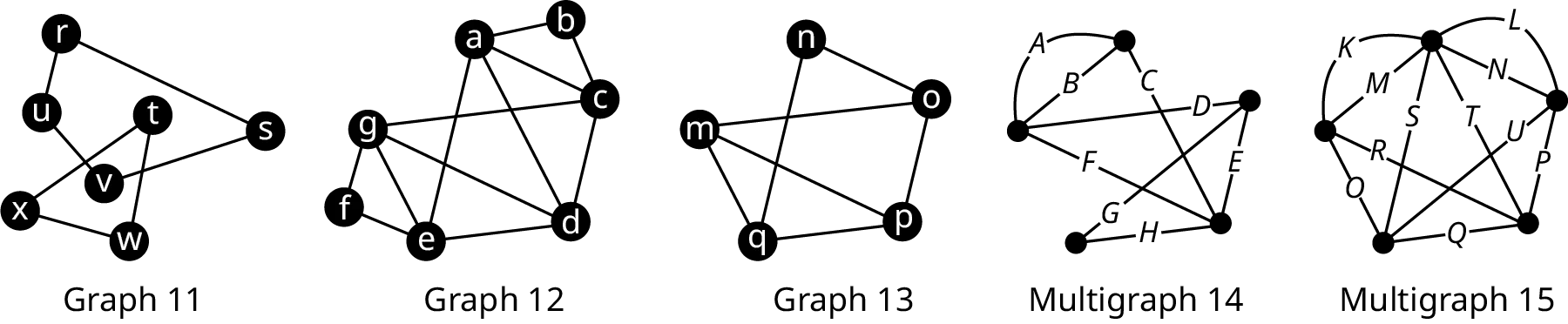34 .
connected
35 .
disconnected
36 .
Eulerian
##### Euler Trails
For the following exercises, use the graphs and multigraphs in Figure 12.354.
37 .
List the set of vertices for each component in Graph 13.
38 .
Determine whether the sequence of edges represents an Euler circuit in Multigraph 15:
KLNMOSTQUPR
39 .
Find an Euler circuit beginning and ending at vertex g in Graph 12 if one exists. Otherwise, explain how you know such an Euler circuit does not exist.
40 .
Give an example of a pair of edges that could be duplicated to eulerize Multigraph 14.
For the following exercises, use the graphs and multigraphs in Figure 12.354. Identify any graphs and/or multigraphs with the given characteristics. If there are none, state so.
41 .
Exactly two vertices of odd degree
42 .
Has an Euler trail
43 .
Has exactly one local bridge
For the following exercises, use the graphs and multigraphs in Figure 12.354. In each exercise a graph and a sequence of vertices are given.
44 .
Determine whether the sequence of edges, ABCHGDFE, is an Euler trail, an Euler circuit, or neither for the graph. If it is neither, explain why.
45 .
Suppose that an edge were added to Graph 11 between vertices s and w. Determine if the graph would have an Euler trail or an Euler circuit, and find one.
##### Hamilton Cycles
For the following exercises, refer to the graph in the given figure.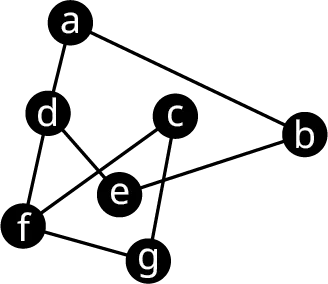46 .
A student has been asked to use Fleury’s algorithm to construct an Euler trail in the given graph. The student decides to begin the trail at vertex d. Is this a good choice, why or why not?
47 .
A student who is using Fleury’s algorithm to construct an Euler trail has decided to begin with fdab → …. If the student is off to a good start, help the student by completing the Euler trail. If the student has made an error, explain the error.
48 .
Use Fleury’s algorithm to construct an Euler trail for Graph 16 beginning at the vertex of your choice.
For the following exercises, use the graphs from the given figures to determine whether the sequence of vertices in the given graph is a Hamilton cycle, an Euler circuit, both, or neither.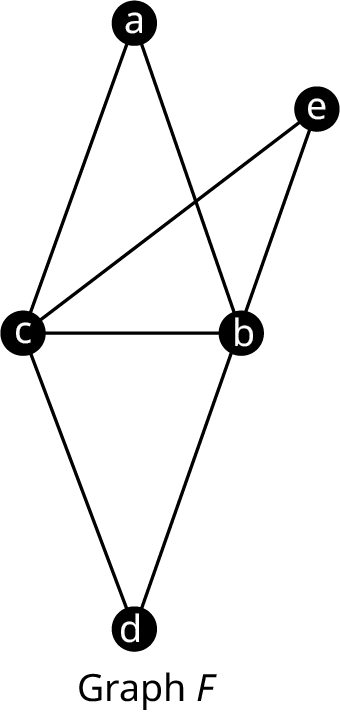49 .
Graph F. abecbdca
50 .
Graph K. mnqopm
51 .
Graph A. bdefcbe
For the following exercises, evaluate the factorial expression for the given value of $n$.
52 .
$\left( {n - 1} \right)!, n = 12$
53 .
$\left( {n - 1} \right)!, n = 14$
54 .
Calculate the number of distinct Hamilton cycles in a complete graph with 13 vertices.
For the following exercises, use the figure shown to find the weight of each Hamilton cycle.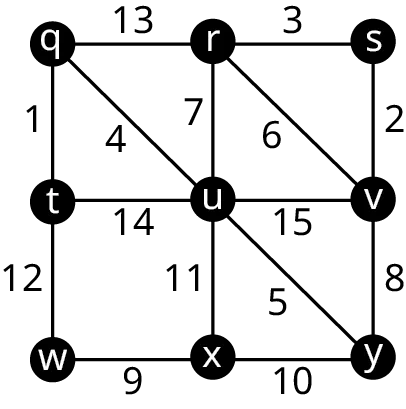55 .
qtwxuyvsrq
56 .
wxyuvsrqtw
##### Hamilton Paths
For the following exercises, use the figure shown to determine whether the sequence of vertices in the given graph is a Hamilton path, an Euler trail, both, or neither.57 .
Graph A. ebcfebe
58 .
Graph A. bcfedbe
59 .
Graph K. nqopm
60 .
Graph K. oqmnp
Recall the three common scenarios in which it is not possible to have a Hamilton between two vertices.
Scenario 1: If an edge ab is a bridge, then there is no Hamilton path between a pair of vertices that are on the same side of edge ab.
Scenario 2: If an edge ab is a bridge with at least three components on each side, then there is no Hamilton path beginning or ending at a or b.
Scenario 3: If a graph is composrd of two cycles joined only at a single vertex p, and v is any vertex that is not adjacent to p, then there are no Hamilton paths beginning or ending at p.
For the following exercises, identify the scenario that guarantees there is no Hamilton path between the given pair of vertices in the given graph.61 .
Vertices a and e.
62 .
Vertices d and f.
The principal of an elementary school plans to visit each classroom exactly once before leaving for the day. The floor plan of the school is given. Use this information for the following exercises.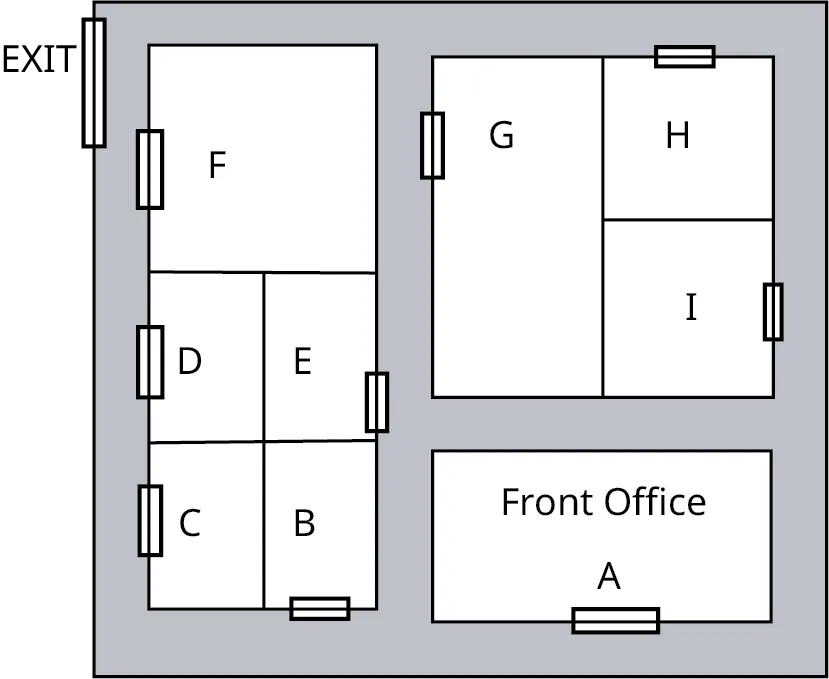63 .
Draw a graph to represent the floor plan for the elementary school in which each vertex is a room and an edge between two vertices indicates that there is a path between the two rooms that does not pass the door to another room.
64 .
Use your graph to find a route that the principal can take beginning at the Front Office in room A, visiting each room exactly once (without passing by another room), and ending at room F, which is next to the exit.
65 .
Would the route you found be best to trace out a Hamilton path, an Euler trail, both, or neither in the graph you drew? Explain how you know.
##### Traveling Salesperson Problem
For the following exercises, determine whether the algorithm described is a greedy algorithm or a brute force algorithm.
66 .
A lumber distributor is loading pallets onto trucks with the intention of using the fewest trucks possible to send a shipment. The distributor loads the pallets with the greatest length that will fit on the truck first and continues loading until no more pallets will fit. Then the next truck is loaded using the same approach.
67 .
A traveler wants to visit five cities by airplane. The traveler lists all the possible orders in which the cities can be visited then calculates the best airfare for each itinerary and selects the least expensive option.
For the following exercises, list all the distinct Hamilton cycles beginning at the given vertex in the given graph. Indicate which pairs of Hamilton cycles are reverses of each other.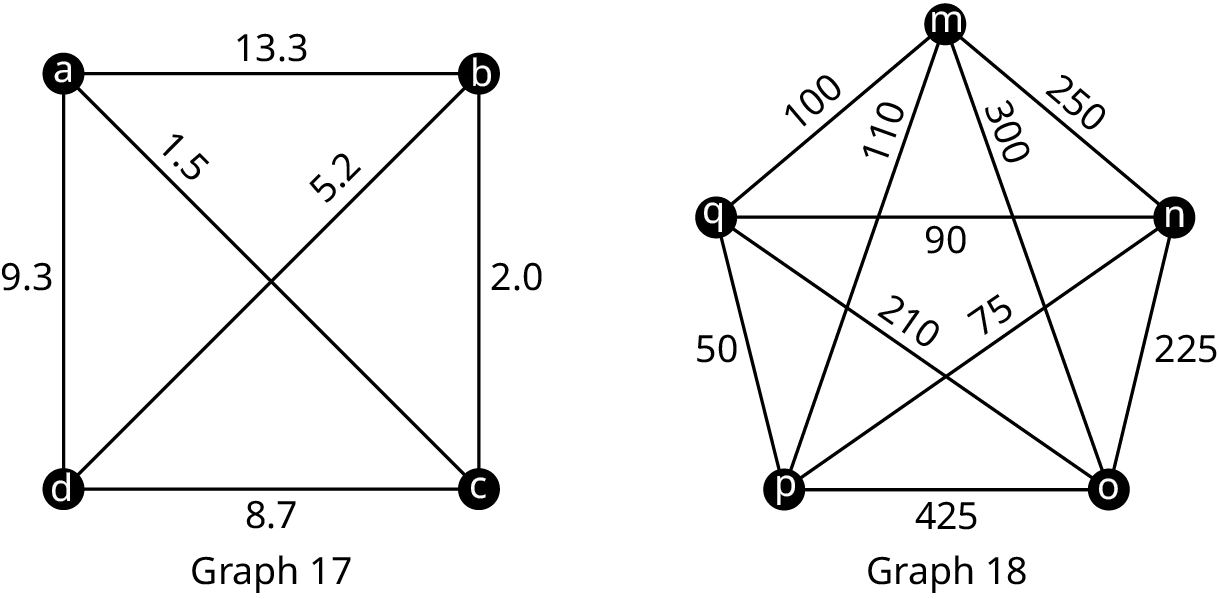68 .
Graph 17, vertex a
69 .
Graph 18, vertex m
For the following exercises, find a Hamilton cycle of least weight for the given graph in Figure 12.362, beginning at the given vertex, and using the brute force method. What is the weight of the cycle?
70 .
Graph 18; vertex m
71 .
Graph 17; vertex a
For the following exercises, find a Hamilton cycle of low weight for the given graph in Figure 12.362, beginning at the given vertex, and using the nearest neighbor method. What is the weight of the cycle?
72 .
Graph 18 vertex m
73 .
Graph 17, vertex a
74 .
The products at a particular factory are manufactured in phases. The same equipment is utilized for each phase, but it must be formatted differently to accomplish different tasks. The transition time to convert between a format for one task and another task varies. The times are given in the table below.

Task A B C D E F G
A $0$ $75$ $130$ $45$ $120$ $70$ $100$
B $75$ $0$ $140$ $65$ $115$ $25$ $60$
C $130$ $140$ $0$ $35$ $55$ $20$ $125$
D $45$ $65$ $35$ $0$ $50$ $30$ $40$
E $120$ $115$ $55$ $50$ $0$ $95$ $145$
F $70$ $25$ $20$ $30$ $95$ $0$ $15$
G $100$ $60$ $125$ $40$ $145$ $15$ $0$
Use the table to draw a graph in which the vertices represent tasks A, C, D, F, and G, and the weights of the edges indicate the times in minutes to transition between pairs of tasks. Use the nearest neighbor method to find an order in which to complete tasks A, C, D, F, and G, which keeps the transition times down and ends with the same set up as it began so that the factory is ready start the next batch. Assume that there are no restrictions on which tasks can be completed in which order. Hint: The nearest neighbor algorithm may give a different result depending on which vertex is the starting vertex; so, you must check all possibilities.
##### Trees
For the following exercises, draw a graph with the given characteristics.
75 .
A tree with eight vertices, exactly two of degree three.
76 .
A connected graph with eight vertices, exactly two of degree 3, which is not a tree.
For the following exercises, identify each type of graph in the given figure: tree graph, star graph, starlike graph, line graph, lobster graph, caterpillar graph, and/or forest graph.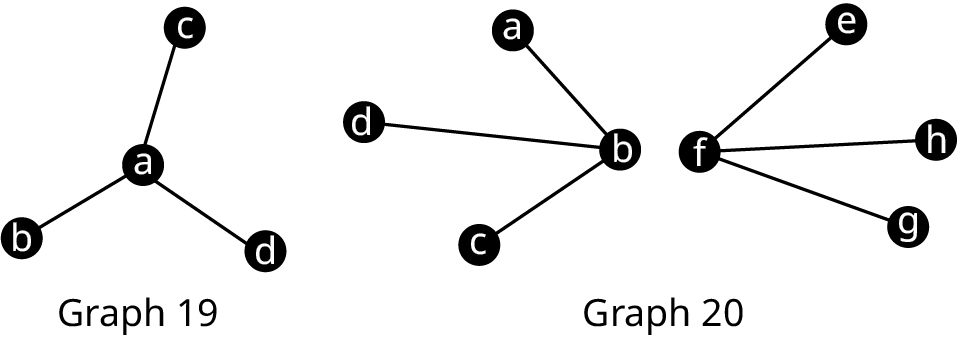77 .
Graph 19
78 .
Graph 20
For the following exercises, use the figure shown to draw three possible spanning trees that fit the given description.79 .
Three spanning trees of Graph A, which include both edges be and de.
80 .
Three spanning trees of Graph K, which include edges mn and oq, but do not include no.
For the following exercises, use Kruskal’s Algorithm to find a minimum spanning tree of the given graph. Graph it and calculate its weight.81 .
Graph 17
82 .
Graph 18
Order a print copy

As an Amazon Associate we earn from qualifying purchases.